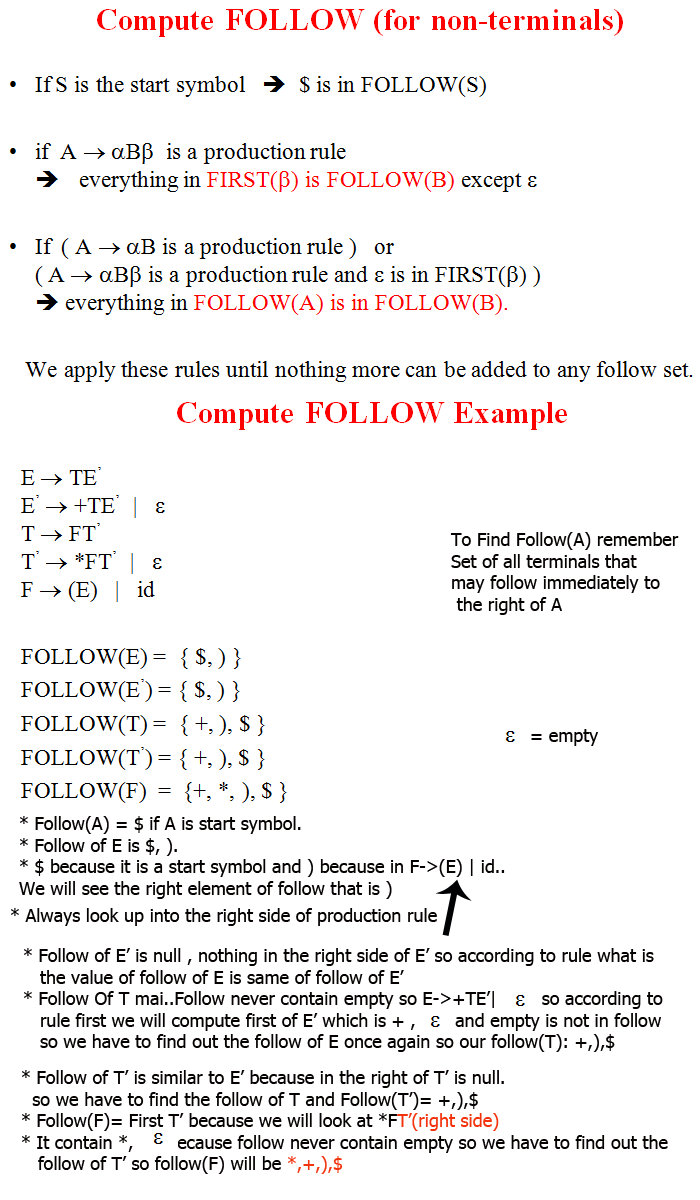## compiler design

It's all about self learning.

Learn First and Follow , Constructing LL(1) parsing tables and Example on First and follow
22 February, 2016

### Construction of LL(1) Parsing table:

• Using First and follow we can construct parsing table.
• Parsing table help us to parse the tree and till now we are directly using the parsing table now we will learn how to construct Parsing table.
• First gives set of all terminals that may derived from alpha.
• If First is a terminal like a,b,(,+,*,id etc..then it is first including empty set element .Eg2: S-> AaAb | BBBa
A-> a
B-> B
Compute First S, A,B ???

Ans: First(S)= AaAb
First of A that is a and first of BbBa that is b
so first (S)= a,b
First(A)= a
First(B)= b
• Follow always find on the right side of the production rule.
• Like we have to find follow(E) so we have to look E->TE.  This E is our element which we need to find.
• Follow(A)=  Set of all terminals that may follow immediately to the right of A.
• like A->Aaaa These are terminals , they are follow of A.
• Follow (A) is \$ if A is start symbol.
• If follow(A) is null or empty set then we will move to left side non-terminal set .
• S->BA.. Find follow(A). So in this case we will find the value of Follow(A) is Follow(S).
• if b is empty set because follow never contain empty set.Que: Find First and follow
S->aBDh
B->cC
C->bC|empty
D->EF
E->g|empty
F->f|empty
Solve the question nai toh move to next chapters so till den have fun bye tc..